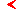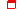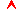﻿ Dynamical chaos - Program of course - Education - Saratov group of theoretical nonlinear dynamicsmainthis page only

# Dynamical chaos

Author: Prof. S.P.Kuznetsov

18 lectureseducationproblems and lessons

## 1. Historical background

Mechanics. Statistical physics. Theory of oscillations, radio-physics and electronics. Hydrodynamics. Iterative mappings. Mathematics. Applied chaos.

## 2. Chaos in artificial dynamical systems

One-dimensional maps. Saw-tooth map. Symbolic dynamics and Bernoulli shift. Logistic map. Variable change of Ulam and von Neumann, developed chaos in the logistic map, and the explicit formula for the chaotic evolution. Iterations in inverse time and RL coding. Tent map.

Two-dimensional area-preserving maps. Baker’s map and its constructing from postulated symbolic dynamics. Visual description of the Baker map and mixing property. Arnold’s cat map. Definition and visual interpretation using picture of the cat face. An infinite set of periodic orbits. Expanding and contracting eigendirections and mixing property.

Strange chaotic attractors. Generalized Baker’s map. Plykin’s attractor. Solenoid of Smale-Williams. Hyperbolicity.

## 3. Lorenz system

Physical problems reducing to the Lorenz equations: Rayleigh-Benard convection, water wheel, convection in the closed loop, one-mode laser, oscillator with inertial nonlinearity.

## 4. Dynamics of the Lorenz system

Results of numerical simulation of the dynamics for the Lorenz model. Intuitive explanation of its chaotic nature with a help of approximate one-dimensional map.

Analytical study of the Lorenz model. Dissipativity of the Lorenz model. Boundedness of the domain occupied by attractor. Fixed points and stability analysis. Bifurcations in the Lorenz model.

## 5. Chaos in realistic models of physical systems: differential equations and iterative mappings

Charts of dynamical regimes.

Discrete time maps. Hénon map and is mechanical model. Ikeda map as a model of nonlinear ring resonator excited by laser. A typical structure in the parameter plane: Crossroad area. Periodically kicked self-oscillator and Zaslavsky map.

Artificially constructed differential equations. Rössler model. Sprott systems.

Driven nonlinear oscillators: Ueda oscillator and LR-diode circuit.

Autonomous electronic self-oscillators. Oscillator of Kijasko-Pikovsky-Rabinovich. Oscillator with inertial nonlinearity of Anishchenko and Astakhov. Oscillator of Dmitriev and Kislov. Chua’s circuit.

## 6. Poincaré cross-section, Smale horseshoe, and Shil’nikov theorem

Poincaré map for autonomous flow systems and stroboscopic map for periodically driven systems: reducing dynamics of three-dimensional systems to two-dimensional mappings.

Smale horseshoe. Constructing the model and explanation of the Cantor-like structure of a set of points surviving in the rectangular domain for asymptotically large number of iterations.

Shil’nikov theorem: appearance of Smale horseshoe and complex dynamics near the situation of presence of loop of separatrix of saddle-focus. Inverse-time version of the theorem.

## 7. Homoclinic structure

Stable and unstable manifolds of saddle fixed point of iterative map and possibility of their intersection. Homoclinic structure. Smale horseshoe and homoclinic structure. Heteroclinic structure.

Mel’nikov’s criterion of appearance of the homoclinic structure in a driven nonlinear oscillator.

## 8. Distribution function, invariant measure, ergodicity, and mixing

Statistical approach to the study of chaos. Ensemble and phase portrait of its dynamics.

Distributions and invariant measures.Krylov-Bogoljubov theorem on existence of an invariant measure. Nonhyperbolic systems and quasiattractors.

Ergodicity and its role for statistical mechanics. Mixing as more strong property necessary to explain relaxation to the statistical equilibrium. Links of mixing with decay of correlations and with high sensitivity to initial conditions.

One-dimensional maps: invariant distributions and Frobenius-Perron equation. Flow systems: equation for the density function and gray scale portraits of strange attractors.

## 9. Stability and instability. Lyapunov exponents

Stability in a sense of Lagrange.

Poisson stability. Wandering and non-wandering points. Limit sets. Definition of the Poisson stability in terms of the limit sets and Poincaré returns. How do the features of Poincaré returns are linked with nature of the dynamical regime (periodic, quasiperiodic, chaotic)?

Lyapunov’s concept of stability and Lyapunov characteristic exponents. Linear stability analysis of an orbit. Geometrical interpretation of the Lyapunov exponents and their algebraic treatment in terms of singular values of the linearized evolution matrix. Lyapunov exponent of fixed points and limit cycles. More general attractors and role of the multiplicative ergodic theorem. General properties of the Lyapunov exponents for autonomous flow systems. Classification of attractors by signature of spectra of Lyapunov exponents.

## 10. Computation of the Lyapunov exponents

Examples of analytical computation of Lyapunov exponents for model maps: saw-tooth map, tent map, generalized Baker’s map.

Numerical approach to computation of the Lyapunov exponents. Using ortogonalization in process of computation of spectrum of the Lyapunov exponents. Examples: logistic map, Hénon map, Ikeda map, Lorenz model, Rössler oscillator.

Two-parameter analysis of nonlinear dynamics and parameter plane charts of Lyapunov exponent.

## 11. Geometry of strange attractors and fractal dimension

Fractal structure of attractor in Rössler oscillator, Hénon map, Smale-Williams model.

Fractals: definition and simple examples. Cantor set, Koch curve, Sierpinski carpet. Example of the Cantor set appearance in one-dimensional iterative map. Measure of the Cantor set and its power (cardinality).

Capacity and box-counting dimension. Dimension of the Cantor set. Hausdorff dimension and its connection with capacity.

Fractal dimension of attractor for generalized Baker’s map

## 12. Generalized dimensions and multi-fractal formalism

Information dimension and its significance. Theorem about fractal dimension of a subset of attractor.

Correlation dimension and algorithm of Grassberger and Procaccia for its computation.

Spectrum of generalized dimensions of Rényi. Definition and examples of computation for generalized Cantor set. Improved definition and dimensions for attractor of generalized Baker’s map. Scaling-spectrum, or singularity spectrum.

Kaplan-Yorke formula and Lyapunov dimension.

## 13. Processing observables: Attractor reconstruction, embedding problem, computation of quantifiers of chaotic dynamics

Time-delay phase space reconstruction. Depicting portraits of attractors and visual estimates.

Numerical computation of the correlation dimension. Estimate of required volume of data suggested by Ecmann and Ruelle.

Embedding theorem and its heuristic explanation.

Method of computation of the Lyapunov exponent from observing time series and estimate of required volume of data.

Idea of reconstruction of dynamical systems from observable time series: research program and its difficulties.

## Bibliography

1. S.P.Kuznetsov. Dynamical chaos, 2001, Moscow, Fizmatlit (in Russian).
2. H.G.Schuster. Deterministic chaos, 1988, Physik-Verlag.
3. P.Bergé, Y.Pomeau, and C.Vidal. Order from Chaos: Towards a deterministic approach to turbulence, 1986, John Wiley&Sons.
4. Strange attractors (Eds. Ya.G.Sinai and L.P.Shil’nikov), 1981, Moscow, Mir (in Russian).
5. F.C.Moon. Chaotic Vibrations, 1986, John Wiley&Sons.
6. M.I.Rabinovich, D.I.Trubetskov. Oscillations and waves in linear and non-linear systems, 1989, Kluver Acad. Publ.
7. L.D.Landau and E.M.Lifshitz. Hydrodynamics.
8. M. J. Feigenbaum. Universal behavior in nonlinear systems. Physica D7, 1983, No 1-3, 16-39.
9. V.S.Anishchenko. Dynamical Chaos - Models and Experiments. World Scientific, Singapore, 1995.
10. Yu.I.Neimark, P.S.Landa. Stochastic and chaotic oscillations, 1987, Moscow, Nauka (in Russian).
11. R.Balescu. Equilibrium and nonequilibrium statistical mechanics, 1975, John Wiley&Sons.
12. M. Schroeder. Fractals, Chaos, Power Laws. Freeman & Co, N.Y., 1991.
13. E.Ott. Chaos in Dynamical Systems. Cambridge Univ.Press, 1993.
14. J.M.T. Thompson and H.B.Stewart. Nonlinear dynamics and chaos. Wiley, N.Y., 1986.
15. C.Beck and F.Schlögl. Thermodynamics of chaotic systems. Cambridge Univ.Press, 1993.
16. J.-P.Eckmann, P.Collet. Iterated Maps as Dynamical Systems, Birkhäuser, Basel, 1980.
17. J. Guckenheimer and P. J. Holmes. Nonlinear oscillations, dynamical systems, and bifurcations of vector fields. Springer-Verlag, Berlin, 1983.
18. B.B.Mandelbrot. The Fractal Geometry of Nature. Freeman, San Francisco, 1982.
19. L.E.Reichl. The Transition to Chaos In Conservative Classical Systems: Quantum Manifistations. Springer-Verlag, N.Y., 1992.maineducationthis page onlytop

Saratov group
of theoretical nonlinear
dynamics